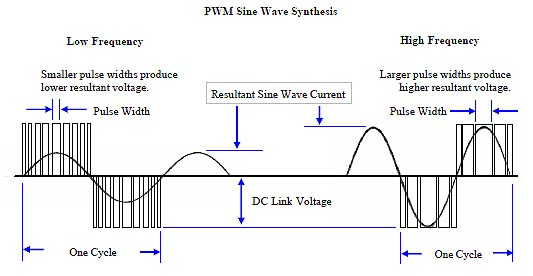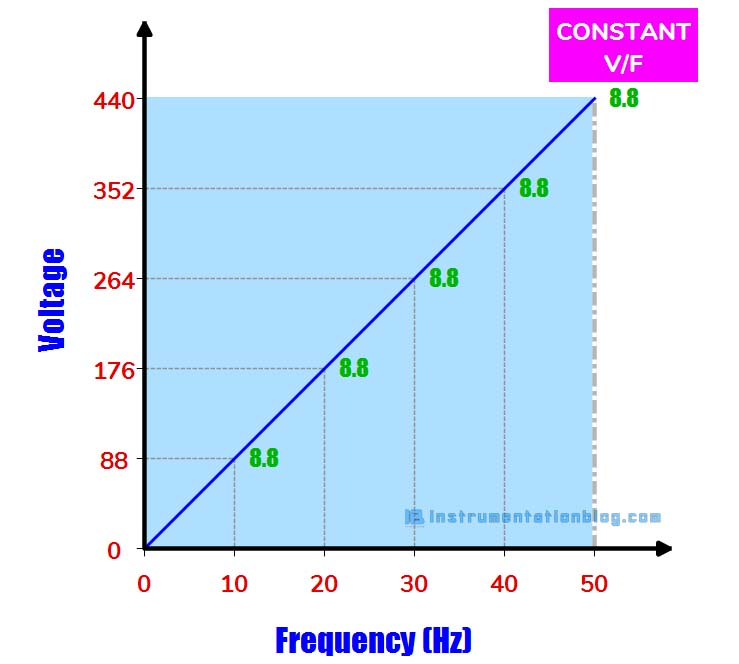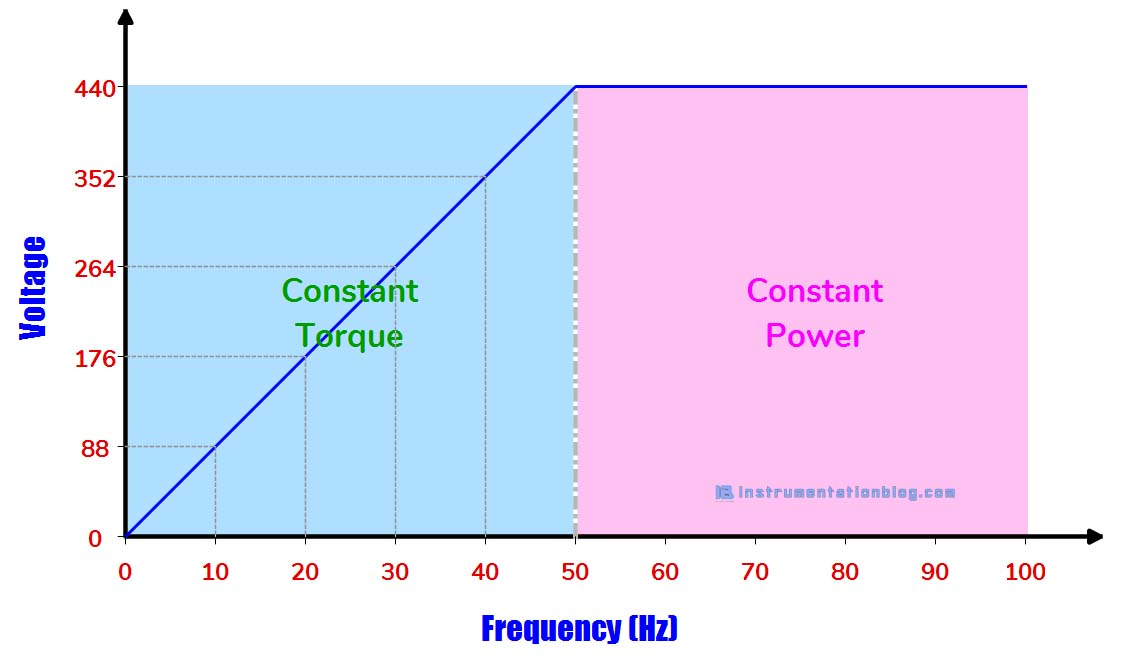# Why voltage to frequency ratio is kept constant in vfd?

## Why Voltage to Frequency ratio is kept constant in VFD?

We all know that VFD means Variable Frequency Drive. VFD is mainly used for induction motor speed control, saving energy, and better process control.

I would like to recommend you to read Basics of an Induction Motor and How a rotating magnetic field works?, before getting the answer of “Why V/F ratio in VFD is kept constant?”.

Motor transforms electrical energy into mechanical energy in the form of motor rotational energy.

When we apply an AC voltage to the motor stator, the rotating magnetic field or magnetic flux is generated. The rotor of the motor works like a magnet that interacts with the generated magnetic field. As the rotating magnetic field rotates, it pulls the rotor and causing it to rotate.

The formula for the generated flux is, Ø = Øm Sin ωt

Faraday’s law of induction states that the EMF induced by a change in magnetic flux is

EMF = – N (dØ/dt)

EMF = – N [d(Øm Sin ωt)/dt]

EMF = – N ω Øm Cos ωt

EMF = N 2 π f Øm Cos ωt

EMF = Em Cos ωt, Here EM = 2 π f N Øm

The induced RMS voltage can be expressed as,

erms = Em / √2

erms = 2 π f N Øm / √2

erms = 4.44 f N Øm

Øm = erms / 4.44 f N

Ø ∝ e / f

Ø ∝ V / F

Thus we can say that the flux generated in the stator depends on the ratio Voltage/Hz.

Now the main question is about the speed of the rotating magnetic field. The factors affecting the speed of the rotating magnetic field is,

Ns = 120 F/P

Where,

• Ns = Synchronous Speed or Rotating Magnetic Field Speed
• F = Frequency
• P = Numbers of Poles

As an example, the synchronous speed for a two-pole motor operated at a frequency of 50Hz is 3000 rpm.

The speed of the motor can be changed by varying the frequency, the voltage needs to be increased or decreased in proportion with the frequency in order to maintain the constant flux ratio.The pulse width modulation of the VFD maintains this constant flux ratio by varying the voltage along with the frequency. Let us take an example of how this v/f ratio is maintained in the VFD,If the motor with a nominal voltage of 440V, the nominal RPM of 1450, and the frequency of 50Hz, Then the flux ratio (V/F) would be (440/50) 8.8 at max voltage and frequency.

When we decrease the speed to 25Hz then the corresponding voltage becomes 220V to maintain the ratio (220/25) 8.8. In this way, PWM maintains the constant V/F ratio in VFD.

What happens when we change the frequency alone without changing the voltage?

When we change the frequency in order to change the speed of the motor without maintaining the voltage then it will generate higher flux. Higher flux will lead to heating in the core of the motor due to increase eddy current losses. As a result, there may be a chance that the insulation of the core will get damaged.

There may be another case in which speed is required above the base speed. In this case, it is not practically possible to increase the voltage above 440V. Therefore it is not possible to maintain a constant voltage to frequency ratio above the motor rated speed.Up to the rated speed, the motor operates in constant torque mode. But above the rated speed, we can’t maintain the constant flux ratio, the flux gets weakens and the motor operates in the constant power mode in this region.